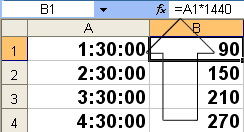### Convert Time to Number (integer)

If you have a time in the format of 1:30:00 but want it to display as the integer 90, here’s the formula.

1. Multiply the time you want to convert to a number by 1440

2. Format the cell as number